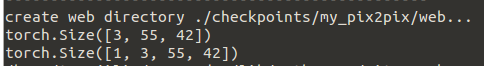My input A is C×H×W. And I want to use torch.nn.Upsample to resize it.
I know the format should be Batch×C×H×W ,so I do:
self.upsample = torch.nn.Upsample(size=[128,128], mode=‘bilinear’)
A = torch.unsqueeze(A, 0) (to get 1×C×H×W)
A = self.upsample(A)
However, it reports:
raise NotImplementedError(“Got 3D input, but bilinear mode needs 4D input”)
NotImplementedError: Got 3D input, but bilinear mode needs 4D input

What is wrong with my code?Can someone help me? Thanks!

Could you check again that you are not passing another `tensor` to `self.upsample`?
This works:

``````A = torch.randn(3, 10, 10)
upsample = nn.Upsample(size=24, mode='bilinear')
A = torch.unsqueeze(A, 0)
A = upsample(A)
print(A.shape)
``````

Sure. Could you print the shape of `patch_up_A_temp` right before the call to `self.upsample`?

thanks,wait one minuteIt seems right. I am confused about it

Seems to be right.
Could you call `self.upsample` outside of the “batch loop”?:

``````x = torch.randn(1, 3, 55, 42)
model.upsample(x)
``````

The size of tensors among the bacth I want to resize are different. For each one I have to resize it respectively. I can not resize them for one batch directly. Any way to solve it?

I’m not sure I understand this issue.
Your `tensors` have a different spatial shape for every batch?
This should not be a problem as `upsample` will reshape it to `[batch, channels, 128, 128]`.

Could you explain the tensor shapes a bit more?

For example, the input is 16×3×128×128 （batchsize=16）.For each one among the batch ，I will crop one patch of different size respectively, that is my patch_up_A_temp. And I want to resize all the patch to the same size(64,64) and finally, for this batch, I get an output of 16×3×64×64.